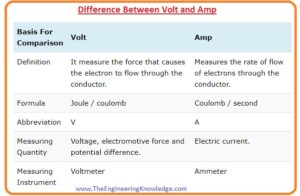Hello, friends, I hope you all are doing great. In today’s tutorial, we will discuss the Difference Between Volt and Amp. The basic difference between volt and amp is that volt is system international unit of voltage, EMF, and potential difference while ampere is a unit of current.

In today’s post, we will have a detailed look at both volt and amp compare them to find their differences. So let’s get started with Difference Between Volt and Amp.

#### Difference Between Volt and Amp

Volt

• Volt is a term that finds the value of force that needed to flow of current in the conductive material.
• The formula of a volt is Joule/coulomb.
• The abbrivation of volt is v.
• Volt is used to measure the quantities Voltage, EMF, and potential difference.
• It can be measured with the voltmeter.

Amp

• Ampere finds the value of the flow rate of electrons in the conductive substance.
• Its formula is Coulomb/second.
• It is the abbreviation of A.
• It used to find the value of the current.
• The ammeter is used to find the value of amp.#### What is Volt

• Volt defines the work done for the movement of electrons from one point to another.
• A volt is also a unit of voltage, electrical potential, and EMF.
• It is denoted with the V. The subunits of volts are mV, uV, kV and MV.
• The definition of a volt is that one joule of work done store charge of one coulomb.#### What is Amp

• The ampere is the system international unit of current. It finds the flow rate of electrons in the conductive material.
• It is denoted with the symbol A. A single ampere is equal to the 1 coulomb of charge that is equal to the  6.242 X 1018 times the elementary charge.#### Comparison between Volt and Amp

• The volt used to find the value of force due to that electron moves in the conductor while amp finds the flow rate of electrons.
• The ration between jouls and coulomb is volt and amp is defined as coulomb per second.
• The symbolic representation of volt is V and amp is denoted by the A.
• Amp is a unit of current and volt is a unit of voltage, EMF, and amp.
• The measuring device of a volt is voltmeter and amp is measured by the ammeter.

It is a detailed post about the difference between volt and amp if you have any further query ask in comments. thanks for reading. Have a good day.